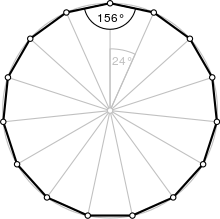Type Regular polygon
Edges and vertices 15
Schläfli symbol {15}
Coxeter diagram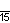Symmetry group Dihedral (D15), order 2×15
Internal angle (degrees) 156°
Dual polygon Self
Properties Convex, cyclic, equilateral, isogonal, isotoxal

In geometry, a pentadecagon or pentakaidecagon or 15-gon is a fifteen-sided polygon.

A regular pentadecagon is represented by Schläfli symbol {15}.

A regular pentadecagon has interior angles of 156°, and with a side length a, has an area given by## Uses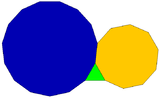A regular triangle, decagon, and pentadecagon can completely fill a plane vertex.

### Construction

As 15 = 3 × 5, a regular pentadecagon is constructible using compass and straightedge: The following constructions of regular pentadecagons with given circumcircle are similar to the illustration of the proposition XVI in Book IV of Euclid's Elements.

Comparison the construction according Euclid in this image: Pentadecagon

In the construction for given circumcircle:is a side of equilateral triangle andis a side of a regular pentagon. The pointdivides the radiusin golden ratio:Compared with the first animation (with green lines) are in the following two images the two circular arcs (for angles 36° and 24°) rotated 90° counterclockwise shown. They do not use the segment, but rather they use segmentas radiusfor the second circular arc (angle 36°).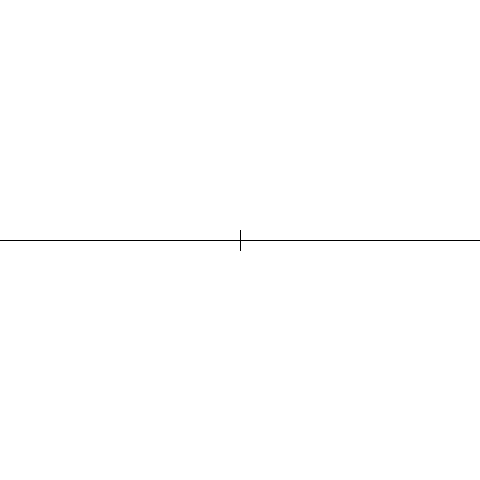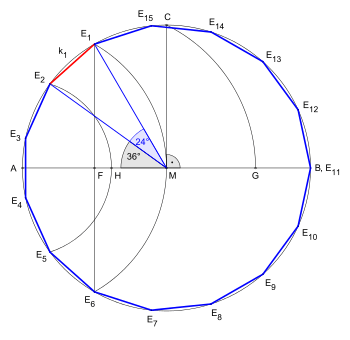A compass and straightedge construction for a given side length. The construction is nearly equal to that of the pentagon at a given side, then also the presentation is succeed by extension one side and it generates a segment, herewhich is divided according to the golden ratio.CircumradiusSideAngle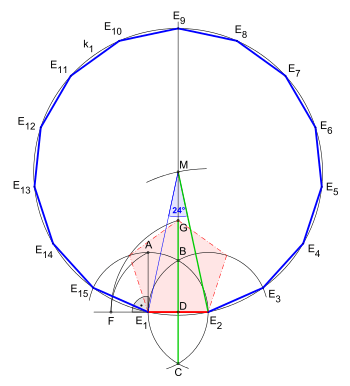Construction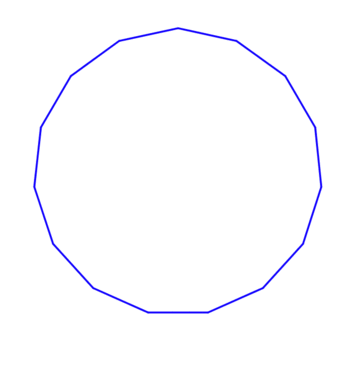Construction as animation

## Symmetry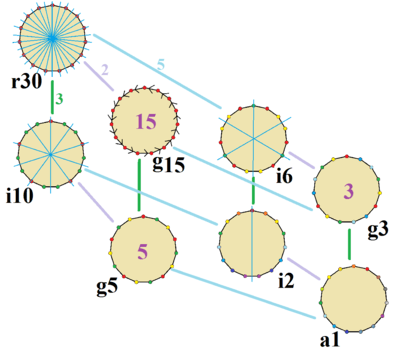The symmetries of a regular pentadecagon as shown with colors on edges and vertices. Lines of reflections are blue. Gyrations are given as numbers in the center. Vertices are colored by their symmetry positions.

The regular pentadecagon has Dih15 dihedral symmetry, order 30, represented by 15 lines of reflection. Dih15 has 3 dihedral subgroups: Dih5, Dih3, and Dih1. And four more cyclic symmetries: Z15, Z5, Z3, and Z1, with Zn representing π/n radian rotational symmetry.

On the pentadecagon, there are 8 distinct symmetries. John Conway labels these symmetries with a letter and order of the symmetry follows the letter. He gives r30 for the full reflective symmetry, Dih15. He gives d (diagonal) with reflection lines through vertices, p with reflection lines through edges (perpendicular), and for the odd-sided pentadecagon i with mirror lines through both vertices and edges, and g for cyclic symmetry. a1 labels no symmetry.

These lower symmetries allows degrees of freedoms in defining irregular pentadecagons. Only the g15 subgroup has no degrees of freedom but can seen as directed edges.

There are 3 regular star polygons: {15/2}, {15/4}, {15/7}, constructed from the same 15 vertices of a regular pentadecagon, but connected by skipping every second, fourth, or seventh vertex respectively.

There are also three regular star figures: {15/3}, {15/5}, {15/6}, the first being a compound of 3 pentagons, the second a compound of 5 equilateral triangles, and the third is a compound of 3 pentagrams.

 Picture Interior angle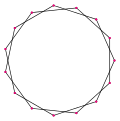{15/2}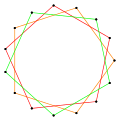{15/3} or 3{5}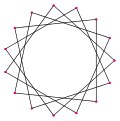{15/4}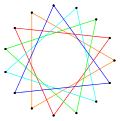{15/5} or 5{3}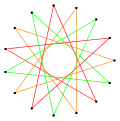{15/6} or 3{5/2}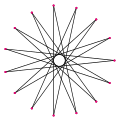{15/7}132° 108° 84° 60° 36° 12°

### Petrie polygons

The regular pentadecagon is the Petrie polygon for some higher-dimensional polytopes, projected in a skew orthogonal projection: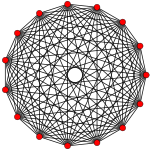14-simplex (14D)

It is also the Petrie polygon for the great 120-cell and grand stellated 120-cell.